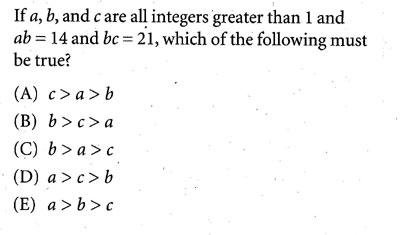Topic : Equation-Diophantine Equation
 from: category_eng
1.2.Integer Property the range of x is limited.

3.Many Variables Eliminate a variable.

 1. e
 2. E Many Variables the range of x is limited.
 3. b Modification Eliminate a variable. a/c = 2/3 let say a=2k and c=3k (k is integer) then bk = 7, b= 7/k but 7/k >1 so.. k<7.. so k=1 b=7, a=2, c=3